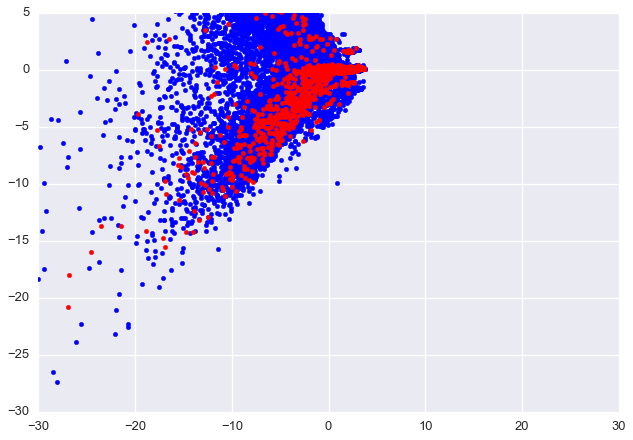# Santander Competition¶

Clay McLeod
Slides @ claymcleod.io/talks

## Overview¶

1. Preprocessing
2. Autoencoders
3. SMOTE
4. Algorithm
5. Results

## Preprocessing¶

Main Problem: Data is high dimensional with a lot of redundant data.

In :
df = pd.read_csv('input/train.csv')
(n_instances, n_features) = df.shape
print('Training data has %d instances with %d features.' % (n_instances, n_features-1))

(n_instances, n_features) = df.shape
print('Testing data has %d instances with %d features.' % (n_instances, n_features))

Training data has 76020 instances with 370 features.
Testing data has 75818 instances with 370 features.


Question: How can we reduce the data's dimensionality?

• Removal of all-zero features (training and testing).
• Scale the data.
• Removal of highly correlated features.
• Perform some kind of dimensionality reduction?
• PCA, ICA, etc. <--- Already has been covered in class
In :
import santander

(X_train, y_train), (X_test, ID_test) = \
dump_data=False, # Cache data
corr=True, # Remove highly correlated features (Pearson)
autoencode=False, # Turn on autoencoding
autoencode_nodes= # Which features to autoencode?
)
(n_instances, n_features) = X_train.shape
print('Training data now has %d features.' % (n_features-1))

  [*] Loading data from CSVs
[*] Extracting training/test data
[*] Removing all features without 5% variance
[*] Scaling input
[*] Dropping highly correlated features
[-] Performing spearman correlation analysis
[-] Dropping 109 highly correlated features
Training data now has 127 features.


Second Problem: Data is highly unbalanced.

In :
negative_class = float(np.count_nonzero(y_train == 0))
positive_class = float(np.count_nonzero(y_train == 1))
ratio = (positive_class / (negative_class + positive_class)) * 100.0
print("Positive class makes up %.2f%% of the data." % (ratio))

Positive class makes up 3.96% of the data.


Question: How can we account for the unbalanced data?

• Ask the oracle for more information (Dr. Chen's suggestion).
• Weigh the cost associated with classes accordingly.
• Undersampling/Oversampling
• Undersampling: Random undersampling, One sided-selection, Condensed Nearest-Neighbor, Near-Miss, Neighborhood Cleaning, Tomek Links.
• Oversampling: Random oversampling, SMOTE, Borderline SMOTE, SVM-based SMOTE.

Third Problem: Data is not discriminative.

Example: Visualization using the first two principle components.

In [ ]:
X_pca = PCA(n_components=2).fit_transform(X_train)
plot_dataset(X_pca, y_train, bounds=[-30, 30, -30, 5])## Autoencoders¶

Idea: Uses neural networks to compress data.

Note: Closesly related to dimensionality reduction</div>

Process

1. Create a neural network with a small number of nodes in the middle layers.
2. Train the network on to recreate the input as the output (reconstruct the data).
3. Take away the second half of the network, leaving the output as the compressed nodes.## SMOTE¶

#### Synthetic Minority Over-sampling Technique¶

Idea: Create synthetic training examples to create a more robust algorithm.

Process

1. Pick a random point in your dataset.
2. Calculate k nearest neighbors.
3. Choose another point randomly from these neigbors.
4. Create a synthetic point somewhere along the vector connecting these two points.
In [ ]:
from unbalanced_dataset import SMOTETomek

STK = SMOTETomek(ratio=1, verbose=True)
stkx, stky = STK.fit_transform(X_train, y_train)
X_pca_stkx = PCA(n_components=2).fit_transform(stkx)
plot_dataset(X_pca_stkx, stky, bounds=[-5, 40, -40, 20])

Determining classes statistics... 2 classes detected: {0: 73012, 1: 3008}

/Users/claymcleod/miniconda2/envs/python2/lib/python2.7/site-packages/unbalanced_dataset/pipeline.py:44: FutureWarning: in the future, boolean array-likes will be handled as a boolean array index
minx = self.x[self.y == self.minc]


## Algorithm¶

Algorithm: eXtreme Gradient Boosting (XGB)

Idea: Create many weak learners to form a strong learner.

Note: XGB is a form of regularized gradient boosting which encourages sparsity and allows greater scalability and consistency.</div>

Process

1. Stratified cross-validation (finding a good cross-validation partition is key).
2. Bayesian Optimization for hyperparameters.
3. Use XGBClassifier with optimized hyperparameters.
In [ ]:
# xgb = XGBClassifier(
#          learning_rate=0.03,
#            n_estimators=350,
#            max_depth=4,
#            min_child_weight=1,
#            gamma=0,
#            subsample=0.8,
#            colsample_bytree=0.8,
#            objective='binary:logistic',